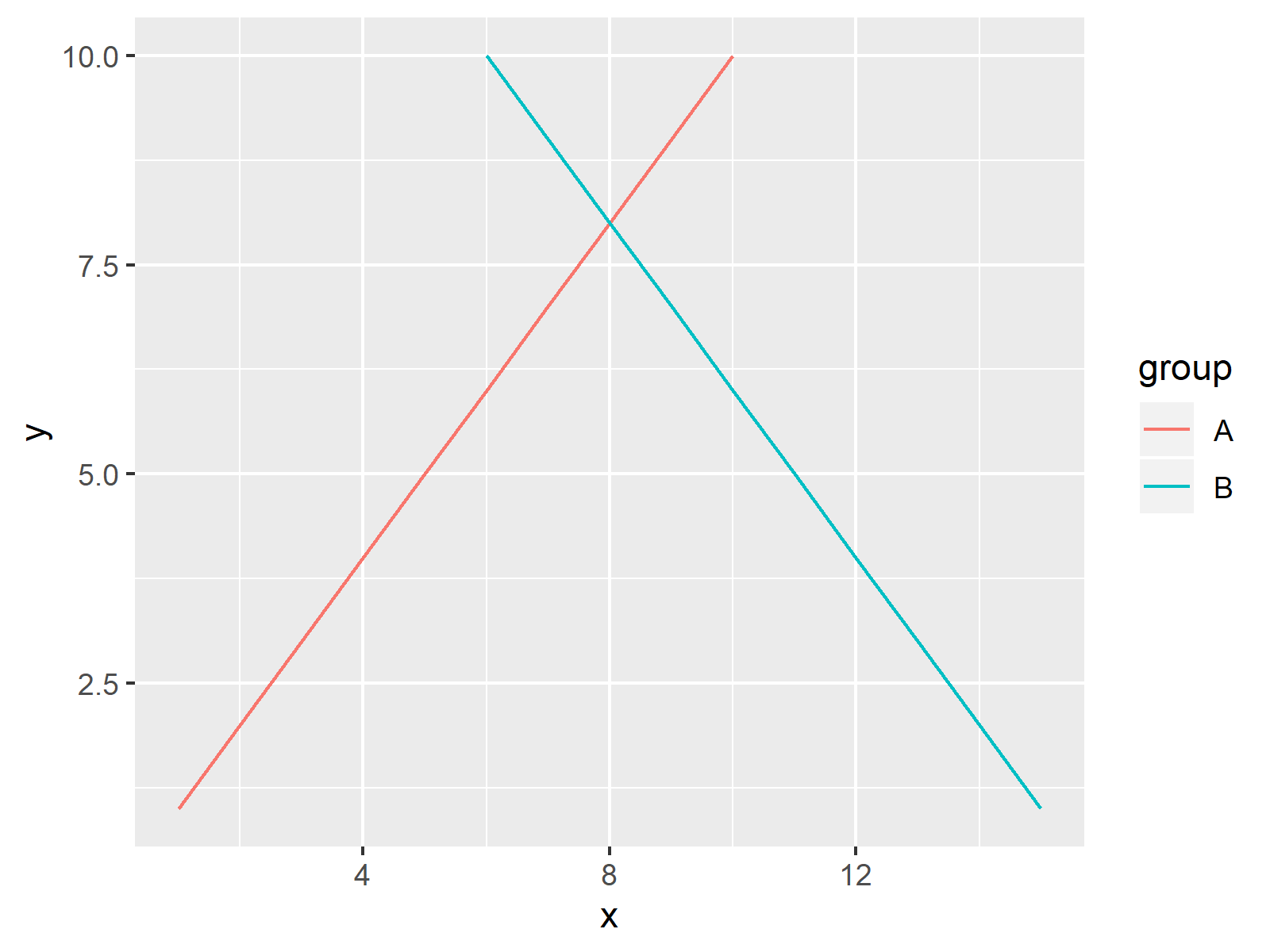# Show ggplot2 Legend at the Bottom of a Plot & Horizontally Aligned in R (Example)

On this page, I’ll explain how to display a ggplot2 legend at the bottom of a plot with horizontal alignment in the R programming language.

The article contains this information:

So now the part you have been waiting for – the R code!

## Construction of Example Data

In this R programming tutorial, we’ll use the following data frame in R:

```data <- data.frame(x = c(1:10, 15:6),                        # Create example data
y = c(1:10, 1:10),
group = c(rep("A", 10), rep("B", 10)))
#   x y group
# 1 1 1     A
# 2 2 2     A
# 3 3 3     A
# 4 4 4     A
# 5 5 5     A
# 6 6 6     A```

Our example data consists of two numeric variables x and y as well as of a grouping variable group.

If we want to draw plots with the ggplot2 package, we need to install and load the package to RStudio:

```install.packages("ggplot2")                                  # Install ggplot2 package

Now, we can create a basic ggplot2 graph with default specifications as follows:

```ggp <- ggplot(data, aes(x = x, y = y, col = group)) +        # Create default ggplot2 line plot
geom_line()
ggp                                                          # Draw ggplot2 line plot```Figure 1: Default ggplot2 Legend on Right Side of Plot.

Figure 1 shows the output of the previous R code. We created a line plot with a legend on the right side of the graphic.

Note that we created a line plot in this example. However, you may also use this example as basement for other types of plots such as scatterplots, histograms, bar charts, and so on.

In the remaining tutorial, I’ll explain how to move this legend to the bottom of the plot with a horizontal alignment. Stay tuned!

## Example: Move ggplot2 Legend to the Bottom of Plot

If we want to display our legend horizontally aligned at the bottom of our graphic, we can use the legend.position argument within the theme function:

`ggp + theme(legend.position = "bottom")                      # Move legend to the bottom`Figure 2: ggplot2 Legend at the Bottom of the Plot with Horizontal Alignment.

Figure 2 displays the result: A ggplot2 plot with a legend at the bottom with horizontal alignment.

## Video & Further Resources

Do you need further explanations on the R programming syntax of this article? Then I can recommend to have a look at the following video of my YouTube channel. In the video, I show the R syntax of this article:

Please accept YouTube cookies to play this video. By accepting you will be accessing content from YouTube, a service provided by an external third party.If you accept this notice, your choice will be saved and the page will refresh.

Furthermore, you could read some of the other tutorials of this homepage:

In summary: In this article, I showed how to change the position of a ggplot2 legend in R programming. Don’t hesitate to let me know in the comments section below, in case you have additional comments and/or questions. Besides that, don’t forget to subscribe to my email newsletter to get updates on the newest tutorials.

Subscribe to the Statistics Globe Newsletter